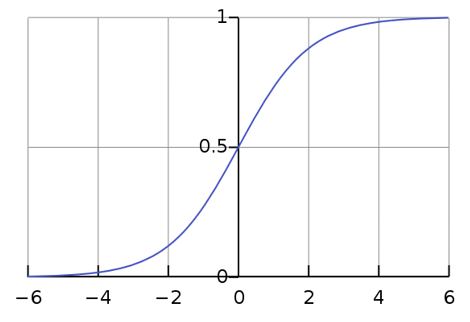/  Technology   /  Regression analysis fundamentals## Regression analysis fundamentals

Regression analysis is a method of predictive modelling technique which infers that there is a relationship between a dependent(target) and independent variable(predictor). Regression analysis is one of the most used and most powerful multivariate statistical techniques. For example, investigation of relationship between effect of price and demand. In this example we consider effect of price as independent variable and demand as dependent variable.

Another example is to find out relationship between age and height of students in a class. In this example height is the dependent variable and age is the independent variable. Saying mathematically,

Height = f(age)

In statistical language it is a simple regression technique, since it has only one predictor and a single target variable. But if there are several predictors and a single target variable. For example, the price of a furniture may depend on type of wood, design, material used and length. Here the price of furniture depends on several factors like type of wood used, design, material used and length. This kind of regression with multiple predictors is defined as multiple regression technique. Mathematically speaking

Price of furniture = f(type of wood used) + f(design) + f(material used) + f(length)

We can also write in the form of regression equation

Price of furniture = x + y1 (#type of wood used) + y2(#design) + y3(#material used) + y4(#length)

The main aim of regression is to find out x, y1, y2, y3, y4.

These simple and multiple regression techniques discussed above are known as linear regression techniques. There are also some non-linear regression techniques available. These regression techniques can be divided into simple and multiple regression techniques which are further divided into linear and non-linear regression techniques.

In machine learning regression is one among many algorithms used to make predictions.

Machine learning problems are generally classified into 1. Classification problems and 2. Regression problems. In classification problem data is labelled i.e., data is assigned a class, for example spam/non-spam or fraud/non-fraud. In regression problem data is labelled with a real value (think floating point).

Let’s us discuss few regression algorithms in machine learning.

#### Regression algorithms in machine learning

In addition to linear regression techniques there are a wide range of algorithms implemented in machine learning. Some of the popular types of regression algorithms are logistic regression, polynomial regression, ridge regression, LASSO, support vector regression, Bayesian Linear Regression.

##### 1.  Logistic Regression

Logistic regression is a type of regression analysis technique, which gets used when the dependent variable is discrete. Example: 0 or 1, true or false, etc. This means the target variable can have only two values, and a sigmoid curve denotes the relation between the target variable and the independent variable.

Logit function is used in Logistic Regression to calculate the relationship between the target variable and independent variables. Below shows the equation that denotes the logistic regression.

logit(p) = ln(p/(1-p)) = b0+b1X1+b2X2+b3X3….+bkXk

where p is the probability of occurrence of the feature.##### 2.  Polynomial Regression

Polynomial Regression is same as multiple regression technique with a slight variation. In Polynomial Regression, the relationship between independent and dependent variables, that is X and Y, is denoted by the n-th degree.##### 3.  Ridge Regression

Ridge Regression is usually used when there is a high correlation between the independent variables. It is a way to decrease model complexity. If there are too many predictors — especially correlated predictors — some predictors are penalized in order to reduce their effects instead of removing them completely.

##### 4.  LASSO

LASSO short for Least Absolute Shrinkage and Selection Operator. It is similar to ridge regression but in LASSO the coefficient value gets nearer to zero. LASSO performs regularization and also feature selection. This feature selection allows only the required features to be selected and making other ones to zero. This helps in preventing overfitting in the model.

##### 5.  Support Vector Regression (SVR)

Support vector machines (SVMs) are the most powerful yet flexible supervised machine learning algorithms which are used for both classification and regression. But usually, they are used in classification problems.  While normal regression reduces errors, SVR fits model such that errors are within a threshold.

##### 6.  Bayesian Linear Regression

Bayesian Linear Regression uses the Bayes theorem to find out the value of regression coefficients. In this method the posterior probability distribution of the features is determined instead of finding the least-squares. Below is the equation for Bayesian Linear Regression.Beyond above regression techniques discussed, there are many other types of regression in machine learning, with Elastic Net Regression, JackKnife Regression, Stepwise Regression, and Ecological Regression.

These different types of regression analysis techniques are helpful to build the model considering the kind of data available or the one that gives the maximum accuracy.